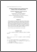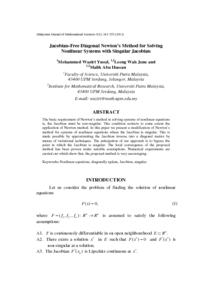# Jacobian-free diagonal Newton's method for solving nonlinear systems with singular Jacobian

## Citation

Yusuf, Mohammed Waziri and Leong, Wah June and Abu Hassan, Malik (2011) Jacobian-free diagonal Newton's method for solving nonlinear systems with singular Jacobian. Malaysian Journal of Mathematical Sciences, 5 (2). pp. 241-255. ISSN 1823-8343

## Abstract

The basic requirement of Newton’s method in solving systems of nonlinear equations is, the Jacobian must be non-singular. This condition restricts to some extent the application of Newton method. In this paper we present a modification of Newton’s method for systems of nonlinear equations where the Jacobian is singular. This is made possible by approximating the Jacobian inverse into a diagonal matrix by means of variational techniques. The anticipation of our approach is to bypass the point in which the Jacobian is singular. The local convergence of the proposed method has been proven under suitable assumptions. Numerical experiments are carried out which show that, the proposed method is very encouraging.Preview
PDF
25078.pdfView Item